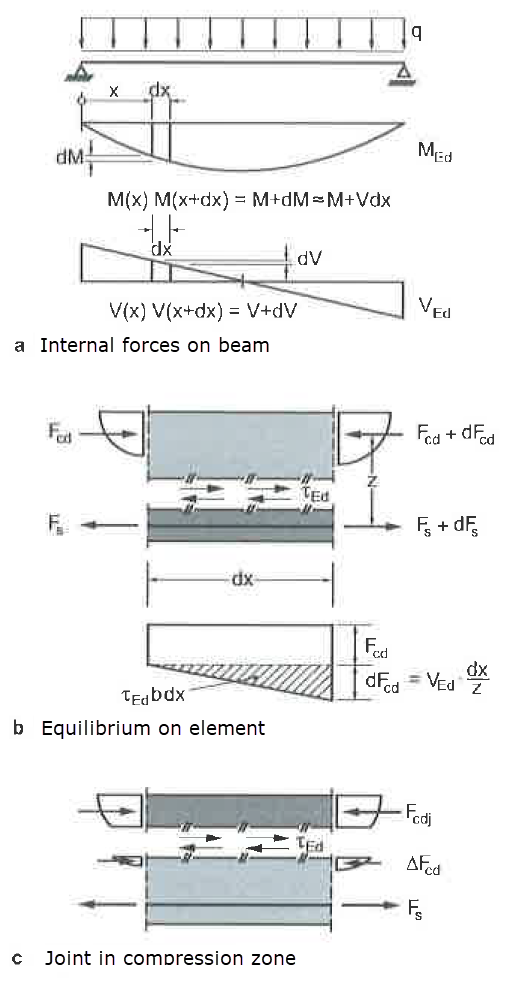# RF-CONCRETE Members – Online Manual Version 5

Online manuals, introductory examples, tutorials, and other documentation.

# 2.1.4 Shear Force Transfer in Joints

### Shear Force Transfer in Joints

When concrete components are added retroactively, the transfer of shear force between the different casting zones must be designed. These so-called shear joints occur for concrete structural components of different ages. In such a case, for example, you need to consider joints between precast parts and cast-in-place concrete additions, or connection joints between construction stages during reconstruction, new construction, or renovation.

The shear force transfer should be designed as follows:

Equation 2.7 EN 1992-1-1, Eq. (6.23)

There are two possibilities for calculating the shear stress in the shear joint:

1. Calculation from Vz,Ed and factor β according to EN 1992-1-1, equation (6.24) without considering Mz,Ed

In this case, vEdi is the design value of the shear force to be resisted per unit of length in the joint. This value is determined by  equation (6.24).

Equation 2.8 EN 1992-1-1, Eq. (6.24)

where

 β quotient from longitudinal force in concrete topping and total longitudinal force in compression or tension zone in considered cross-section VEd design value of applied shear force z lever arm of combined cross-section bi width of joint

The design value of the shear resistance vRdi is determined by the following equation:

Equation 2.9 EN 1992-1-1, Eq. (6.25)

where

 c, μ coefficients that depend on roughness of joint according to  6.2.5 (2) fctd design value of concrete tensile strength according to  3.1.6 (2)P σn smallest stress perpendicular to joint, which acts simultaneously with shear force (positive for compression) where σn < 0.6 ⋅ fcd ρ As / Aiwhere As cross-sectional area of reinforcement crossing the joint Ai area of connection joint α inclination angle of joint reinforcement ν strength reduction factor according to  6.2.2 (6)

2. Calculation from difference of axial forces in added concrete part from general stress integration

The rigid bond assumed for the design of shear joints in the ULS should be primarily reached by an adhesive bond, that is, adhesion and micromechanical gearing. Hence, the joint reinforcement is responsible for the transfer of forces after overcoming the rigid bond, as well as for the ductility of the connection, while the shear joint would have to be designed solely for the adhesive bond.

In the current standards, this approach is accommodated only to a minor degree. Though a movable bond is allowed, it is conservatively differentiated and complemented by construction rules, to be on the safe side.

For shear joints, which are designed for the ultimate limit state for a moveable bond as planned, serviceability limit state designs must additionally be performed. For this case, the moveable bond must be consistently included in the determination of the internal forces and stresses in the ULS and SLS.

Self-equilibrating stresses normally involving shear stresses in the joint (due to the varying shrinkage behavior of two concrete components of different ages, for example) are generally not considered. The acting shear force vEdi is solely calculated from internal forces on the cross-section.Figure 2.2 Shear stresses in joints according to 

Figure 2.2 shows a section of the length dx from a beam with a shear joint parallel to the structural component's axis. The variable bending moment causes a change in the flange forces along the length. For example, the following applies to the compression flange:

There is an equilibrium between the compression force change and the shear stresses in the joint.

Thus, for a constant lever arm z, the stress of the shear joint is in proportion to the shear force VEd, with a constant axial force having no influence on the shear force in the joint parallel to the component axis.

If the shear joint lies within the compression zone, only the portion of the flange force difference between joint and compression flange edge must be transferred. As a result, τEd becomes:

Literatur
  Eurocode 2: Design of concrete structures - Part 1-1: General rules and rules for buildings; EN 1992-1-1:2011-01  Zilch, Konrad u. Zehetmaier, Gerhard. Bemessung im konstruktiven Betonbau. Springer Verlag, 2. Auflage 2010